# 5_6203803968120817053.pptx

May. 28, 2023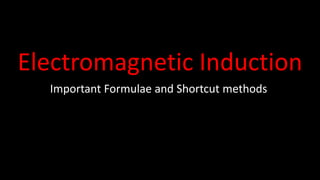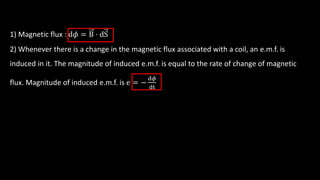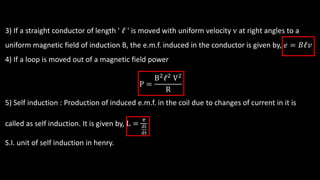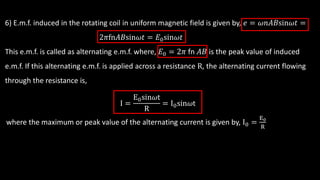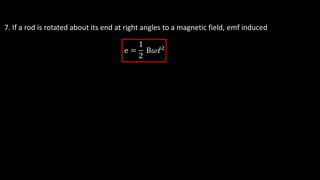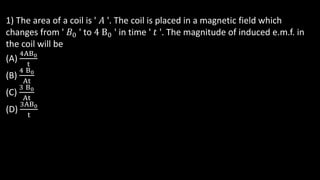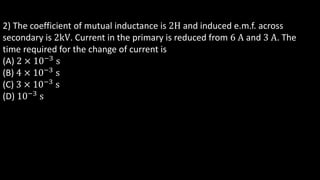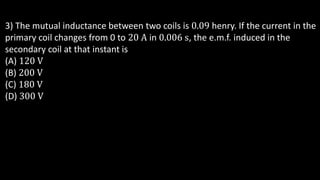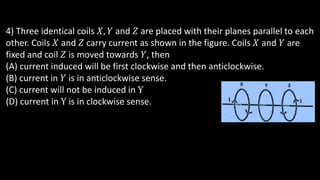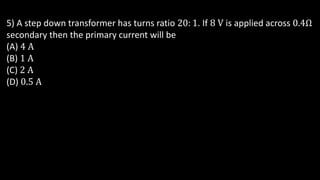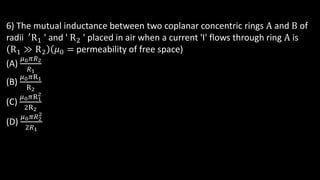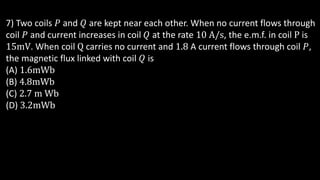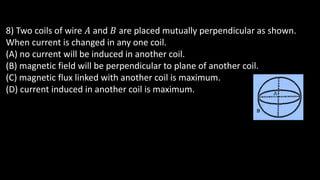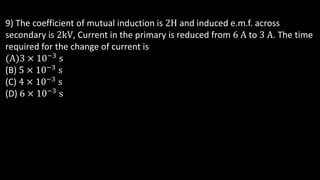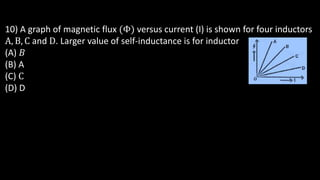1 of 16

### 5_6203803968120817053.pptx

• 1. Electromagnetic Induction Important Formulae and Shortcut methods
• 2. 1) Magnetic flux : d𝜙 = B ⋅ dS 2) Whenever there is a change in the magnetic flux associated with a coil, an e.m.f. is induced in it. The magnitude of induced e.m.f. is equal to the rate of change of magnetic flux. Magnitude of induced e.m.f. is e = − d𝜙 dt
• 3. 3) If a straight conductor of length ' 𝓁 ' is moved with uniform velocity v at right angles to a uniform magnetic field of induction B, the e.m.f. induced in the conductor is given by, 𝑒 = 𝐵𝓁𝑣 4) If a loop is moved out of a magnetic field power P = B2𝓁2 V2 R 5) Self induction : Production of induced e.m.f. in the coil due to changes of current in it is called as self induction. It is given by, L = e di dt S.I. unit of self induction in henry.
• 4. 6) E.m.f. induced in the rotating coil in uniform magnetic field is given by, 𝑒 = 𝜔𝑛𝐴𝐵sin𝜔𝑡 = 2𝜋fn𝐴𝐵sin𝜔𝑡 = 𝐸0sin𝜔𝑡 This e.m.f. is called as alternating e.m.f. where, 𝐸0 = 2𝜋 fn 𝐴𝐵 is the peak value of induced e.m.f. If this alternating e.m.f. is applied across a resistance R, the alternating current flowing through the resistance is, I = E0sin𝜔t R = I0sin𝜔t where the maximum or peak value of the alternating current is given by, I0 = E0 R
• 5. 7. If a rod is rotated about its end at right angles to a magnetic field, emf induced e = 1 2 B𝜔𝓁2
• 7. 1) The area of a coil is ' 𝐴 '. The coil is placed in a magnetic field which changes from ' 𝐵0 ' to 4 B0 ' in time ' 𝑡 '. The magnitude of induced e.m.f. in the coil will be (A) 4AB0 t (B) 4 B0 At (C) 3 B0 At (D) 3AB0 t
• 8. 2) The coefficient of mutual inductance is 2H and induced e.m.f. across secondary is 2kV. Current in the primary is reduced from 6 A and 3 A. The time required for the change of current is (A) 2 × 10−3 s (B) 4 × 10−3 s (C) 3 × 10−3 s (D) 10−3 s
• 9. 3) The mutual inductance between two coils is 0.09 henry. If the current in the primary coil changes from 0 to 20 A in 0.006 s, the e.m.f. induced in the secondary coil at that instant is (A) 120 V (B) 200 V (C) 180 V (D) 300 V
• 10. 4) Three identical coils 𝑋, 𝑌 and 𝑍 are placed with their planes parallel to each other. Coils 𝑋 and 𝑍 carry current as shown in the figure. Coils 𝑋 and 𝑌 are fixed and coil 𝑍 is moved towards 𝑌, then (A) current induced will be first clockwise and then anticlockwise. (B) current in 𝑌 is in anticlockwise sense. (C) current will not be induced in Y (D) current in Y is in clockwise sense.
• 11. 5) A step down transformer has turns ratio 20: 1. If 8 V is applied across 0.4Ω secondary then the primary current will be (A) 4 A (B) 1 A (C) 2 A (D) 0.5 A
• 12. 6) The mutual inductance between two coplanar concentric rings A and B of radii ′ R1 ' and ' R2 ' placed in air when a current 'I' flows through ring A is R1 ≫ R2 𝜇0 = permeability of free space) (A) 𝜇0𝜋𝑅2 𝑅1 (B) 𝜇0𝜋R1 R2 (C) 𝜇0𝜋R1 2 2R2 (D) 𝜇0𝜋𝑅2 2 2𝑅1
• 13. 7) Two coils 𝑃 and 𝑄 are kept near each other. When no current flows through coil 𝑃 and current increases in coil 𝑄 at the rate 10 A/s, the e.m.f. in coil P is 15mV. When coil Q carries no current and 1.8 A current flows through coil 𝑃, the magnetic flux linked with coil 𝑄 is (A) 1.6mWb (B) 4.8mWb (C) 2.7 m Wb (D) 3.2mWb
• 14. 8) Two coils of wire 𝐴 and 𝐵 are placed mutually perpendicular as shown. When current is changed in any one coil. (A) no current will be induced in another coil. (B) magnetic field will be perpendicular to plane of another coil. (C) magnetic flux linked with another coil is maximum. (D) current induced in another coil is maximum.
• 15. 9) The coefficient of mutual induction is 2H and induced e.m.f. across secondary is 2kV, Current in the primary is reduced from 6 A to 3 A. The time required for the change of current is A)3 × 10−3 s (B) 5 × 10−3 s (C) 4 × 10−3 s (D) 6 × 10−3 s
• 16. 10) A graph of magnetic flux Φ) versus current (I) is shown for four inductors A, B, C and D. Larger value of self-inductance is for inductor (A) 𝐵 (B) A (C) C (D) D